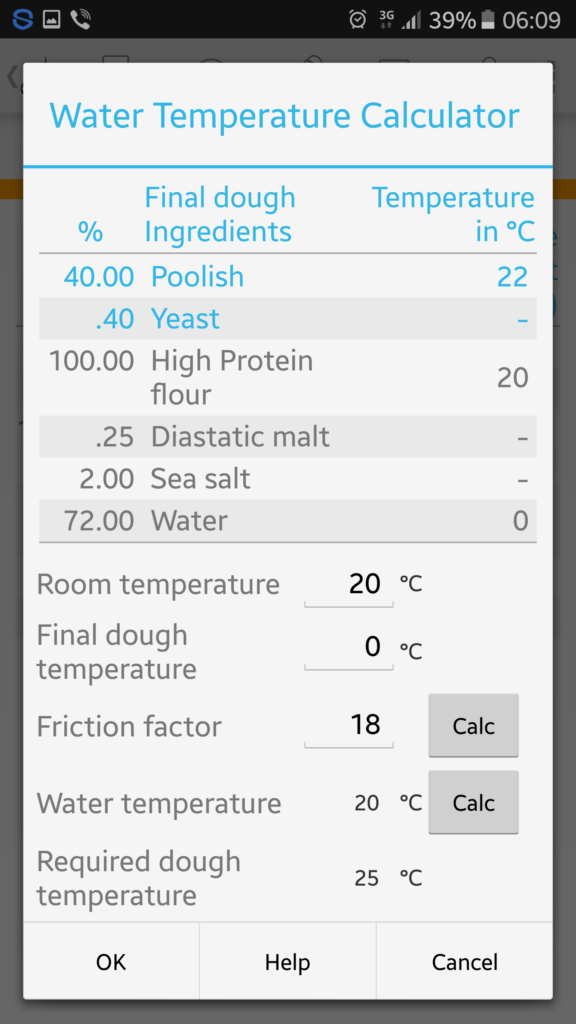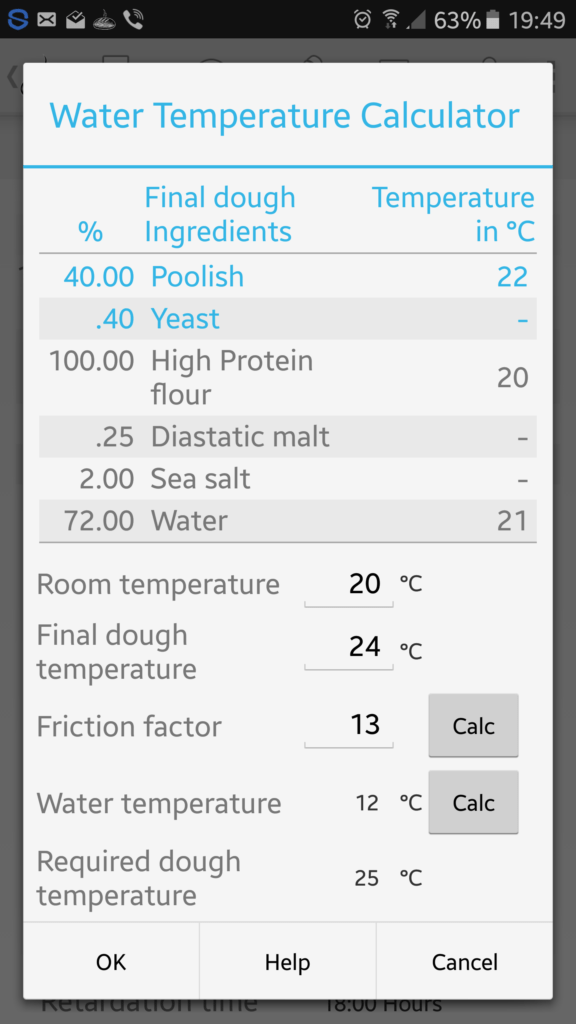Posted by on Dec 1, 2016 in Baking Tips, Bread Boss, slider | 0 comments# What temperature should your water be?

Good bread is born of controlling the key factors that influence bread quality. These are physical processes through micro-organism activity. After all, micro-organisms do all the work converting ‘grain into bread’!

Temperature is one of those key factors that bakers need to consider and use to influence the condition of their sourdoughs and bread doughs. As micro-organisms are highly dependent on their environment we want to get as close to giving them the best conditions as possible for them, our schedules and not least of all bread flavour and quality! The rate of bacterial and yeast multiplication, metabolic activity and enzyme activity is dramatically influenced by temperature. So we need to get it as close to optimum as we can.

Earlier I wrote up about the Major Factor (MF) method for calculating the water temperature for bread dough making. In this post I’d like to cover the “Base Temperature” (BT) method of calculating dough water temperature. So why another method? The reason is that the Base Temperature method has more accuracy when using pre-ferments, sourdoughs or quantities of other ingredients with varying temperatures, such as refrigerated ingredients.

Professional bakers usually have a tight schedule as empty ovens are the bane of bakers who make a living from their trade. Since time is money during any manufacturing process,  dough scheduling is critical. But considering the quality of their product, scheduling is also critical.

At home it’s less important as we can always wait a little, have another cup of coffee or do something else that takes our fancy until our dough is at optimum maturity. But having said that, it’s better to know how to prevent leads and lags in time by getting as close as possible to the temperature we require.

## Base Temperature (BT) Method

The Base Temperature (BT) method includes the following temperatures with the addition of the ‘friction factor’:

• Flour Temperature
• Room Temperature
• Required Water Temperature
• Friction Factor

As with all mixing of bread dough, temperature is generated by friction. This friction, be it low, during hand mixing, or higher, during machine mixing, has an influence on the Finished Dough Temperature (FDT).

The first three temperatures are easily measured but the Friction Factor (FF) needs to be established. To establish the Friction Factor (FF) for the type of mixing we are going to use, we need to employ a test case. This Friction Factor (FF) is then used in the Base Temperature calculations (BT).

### Establishing the Friction Factor (FF)

Here is the method for calculating the friction factor (FF). Prior to making the dough we note the following temperatures:

1. Flour Temperature (FT)
2. Room Temperature (RT)
3. Water Temperature (WT)

We then proceed to make the dough as we normally would. When the dough is fully developed, as we require, we note the Finished Dough Temperature (FDT).

Since we have noted three (3) temperatures that were influential, we need to multiply the Finished Dough Temperature (FDT) by 3 to introduce symmetry to the calculation.

```FDT (Finished Dough Temperature) = 24°C x 3 = 72

FT (Flour Temperature) = 20
RT (Room Temperature)  = 20
WT (Water Temperature) = 13
Total                  = 53```

To finally determine the Friction Factor (FF) we subtract the total of the Finished Dough Temperature (FDT) x 3 or in this example ~  72 from the total of the three temperatures we noted – in this case ~ 53

`72 - 53  = 19`

Our Friction Factor (FF) is 19.

Note: Of course this factor can vary depending on the level of hydration and the types of dough we are making. A ‘slack’ or ‘wet’ dough will mean less friction which produces less heat compared to a ‘tighter’ dough. As with everything in baking the more variables introduced in our scenario a need arises to reestablish the relevant Friction Factor. So we may have several that are to be applied to varying dough types. This is something you should keep on file with each of your formulas (more on this later).

So let’s go further and apply all this to another example, so we can illustrate how the above information can be used to determine our Required Water Temperature (RWT) for a dough, so that we can achieve the Finished Dough Temperature (FDT) required. After all, knowing our Required Water Temperature (RWT) to achieve a desired Finished Dough Temperature (FDT) is the reason we are establishing the Friction Factor (FF).

### Example

We’ve recorded the following:

```Flour Temperature (FT) = 20
Room Temperature (RT)  = 20
Friction Factor (FF)   = 19

TOTAL                  = 59```
`Required Dough Temperature (RDT) = 24 x 3 = 72`

Now calculate the following:

`72 - 59 = RWT of 13°C`

So now we have our Required Water Temperature of ~ 13°C to make our bread dough to achieve a Finished Dough Temperature of 24ºC.

### More utility

To give this method more utility we can take into account the temperature of a sponge/pre-ferment (PF) or a sourdough (SD) also. We would do this by recording its temperature and including it in our calculations. See below:

```FT    = 20
RT    = 20
FF    = 19
SD    = 21
TOTAL = 80```

However, now that we have 4 temperatures we will need to multiply our Required Dough Temperature (RDT) four (4) times to maintain the calculation symmetry.

`Required Dough Temperature (RDT) = 24 x 4 = 96`

Now calculate the following:

`96 - 80 = RWT of 16°C`

Of course any number of large ingredient portions we wish to add to dough can be taken into account this same way. For instance; when making brioche we use a large portion of eggs which may or may not be refrigerated. The only thing we must do is keep the equation symmetrical by multiplying the Required Dough Temperature (RDT) the same number of times as temperatures we have added to our list.

This isn’t complicated and shouldn’t be intimidating, in fact it’s dead easy!

I’m glad to let Bread Boss users know that the recent updated version of Bread Boss lets you determine your Required Water Temperature (RWT) and your Friction Factor (FF) by recording a few simple temperatures and entering them in the fields provided.Shows the field to click which opens the water temperature calculator.

Here’s the calculator below which opens when the “Use Water Temperature” field is clicked in the app.Screen shot from Bread Boss showing the fields for water temperature calculations for the final bread dough

As I mentioned earlier, bread boss formulas will also allow you to keep on file the relevant Friction Factor (FF) for a given dough.

However, first Bread Boss gives you the ability to calculate the Friction Factor (FF) so it can be applied to the Required Water Temperature (RWT) calculations. It also has the flexibility to allow you to enter a Friction Factor of your own choosing. This is an invaluable feature for those who already know the friction factor for their specific dough.Showing the FF calculation when the water temperature is chosen. This also allows the user to enter the Final Dough Temperature as the dough is made then its temperature measured so Bread Boss can calculate the FF.